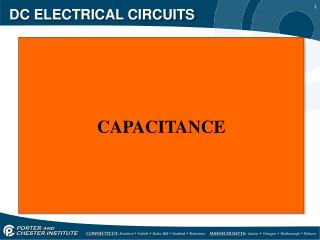DownloadDownload PresentationDC ELECTRICAL CIRCUITS

# DC ELECTRICAL CIRCUITS

Télécharger la présentation## DC ELECTRICAL CIRCUITS

- - - - - - - - - - - - - - - - - - - - - - - - - - - E N D - - - - - - - - - - - - - - - - - - - - - - - - - - -
##### Presentation Transcript

1. DC ELECTRICAL CIRCUITS CAPACITANCE

2. DC ELECTRICAL CIRCUITS • Capacitance is the property of a circuit or device that enables it to store electrical energy by means of an electrostatic field. • A capacitor has the ability to store electrons and release them at a later time, the number of electrons a capacitor can store is determined by it’s capacitance.

3. DC ELECTRICAL CIRCUITS • Capacitor construction consists of two metal plates with a dielectric (non-conducting material) in the middle.

4. DC ELECTRICAL CIRCUITS • Different types of capacitors;

5. DC ELECTRICAL CIRCUITS • The capacitor charges until the difference of potential between the two plates is the same as the applied voltage, current does not flow through the capacitor.

6. DC ELECTRICAL CIRCUITS • Discharging the capacitor occurs when the switch arm is removed from the battery side and to the other branch, electrons move from the negative plate through the circuit to the positive plate until it is completely discharged.

7. DC ELECTRICAL CIRCUITS • Capacitance is measured in farads (f). • One farad is the amount of capacitance that will store a charge of one coulomb of electrons when an EMF of one volt is applied. • A farad is an extremely large value of capacitance, so the unit microfarad is commonly used (uf).

8. DC ELECTRICAL CIRCUITS • Factors that determine capacitance are the area of the plates, the spacing between plates and the dielectric material. • Variable capacitors use moving plates to vary the capacitive value.

9. DC ELECTRICAL CIRCUITS • Fixed capacitors are often named for the material used as their dielectric; Paper Ceramic Mica Electrolytic

10. DC ELECTRICAL CIRCUITS • One of the most popular capacitors is the electrolytic capacitor. • The electrolytic capacitor is polarized, it has a positive and negative lead which means the polarity must be maintained when its used in a circuit. THE STRIPE WITH THE (-) SYMBOL INDICATES THE NEGATIVE LEAD

11. DC ELECTRICAL CIRCUITS • If polarity is not maintained with an electrolytic capacitor it could result in the capacitor exploding.

12. DC ELECTRICAL CIRCUITS • Capacitors have a voltage rating that indicates the maximum voltage that the capacitor can withstand without the dielectric breaking down or arcing over.

13. DC ELECTRICAL CIRCUITS • There are two factors that determine the charge and discharge time of a capacitor. • They are the capacitance of the capacitor and the value of the resistance through which the capacitor must charge or discharge. • The RC time constant is the time required for a capacitor to charge to 63.2% of the applied voltage.

14. DC ELECTRICAL CIRCUITS • The time constant formula is; T = R x C (R= resistance in ohms and C = capacitance in farads). If C= 1uf and R= 100Ωthen; T = R x C 100Ω x 1uf = 100uS (micro seconds) • At this point the capacitor has charged to 63.2% of the applied voltage in the first time constant.

15. DC ELECTRICAL CIRCUITS • The circuit below has 100V applied, the capacitor will charge to this applied voltage, however it will not happen instantaneously.

16. DC ELECTRICAL CIRCUITS • The time constant for the circuit is T = R x C or 1MΩx 1uf; 1,000,000 x .000001 = 1 second. • 100V – 63.2 = 36.8V (voltage remaining after first time constant) • 63.2% of the remaining voltage; 36.8 x .632 = 23.3V. • 36.8V + 23.3V = 86.5V (the cap is charged to 86.5V)

17. DC ELECTRICAL CIRCUITS • It will take about 5 time constants for the capacitor to fully charge, just like an inductor. • Because it took 1 second for the time constant, it will take 5 seconds for the capacitor to fully charge. • It will take the capacitor 5 seconds to discharge as well.